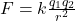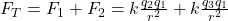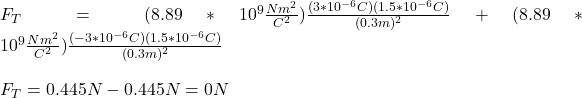## Three charges 1.5*10-6, 3*10-6, -3*10-6 are placed at three vertices of an equilateral triangle of side 30cm. Find the net force acting on 1

Question

Three charges 1.5*10-6, 3*10-6, -3*10-6 are placed at three vertices of an equilateral triangle of side 30cm. Find the net force acting on 1.5*10-6 due to the other two

in progress 0
7 months 2021-07-30T15:42:22+00:00 2 Answers 2 views 0

## Answers ( )

Explanation:

Fnet =F12 + F13

Therefore,

The force of attraction between two charges is given as

F12 = kq1q2/r²

F12=9×10^9×1.5 ×10^-6×3×10^-6/0.3²

F12 = 0.45N

Also,

F13 = kq1q3/r²

F13=9×10^9×1.5 ×10^-6×3×10^-6/0.3²

F13 = 0.45N

From the diagram we notice that F12 is acting between positive x-axis and y axis

F12 = F12Sin30 •i + F12 Cos30 •j

F12 = 0.45Sin30 •i + 0.45Cos30 •j

F12 = 0.225 •i + 0.39 •j N

F13 is acting between positive x axis and negative y axis

Then,

F13 = F12Sin30 •i — F12 Cos30 •j

F13 = 0.45Sin30 •i — 0.45Cos30 •j

F13 = 0.225 •i — 0.39 •j N

Then,

Fnet = F12 + F13

Fnet = 0.225 •i + 0.39 •j + N0.225 •i – 0.39 •j N

Fnet = 0.45 •i N

The net force has a magnitude of 0.45N and it is acting on the positive x axis

Then it direction is

X = arctan(y/x)

X = arctan(0)

X = 0°

F = 0N

Explanation:

The force between two charges is given bywhere r is the distance between the charges and K is the Coulomb’s constant

(k=8-89*10^9Nm^2/C^2)

The force in the first charge is only the sum of the forces due to the other charges. Hence we haveFt=0N

Hope this helps!!